output.to from Sideway
Trigonometry

Angle and Trig. Functions

Draft for Information Only

Content

Trigonometry
Angles
Measure of Angle
Degree Measure of Angle
Angles on Coordinate Plane
Right-Angled Triangle
Trigonometric Functions
Pythagorean theorem
Values of Trigonometric Functions
Table of Trigonometric Functions for Common Angles
More Values of Trigonometric Functions

Trigonometry

Trigonometry is the study of angles and the angular relationship of triangular figures. Although the relationship between an angle and the ratio of sides in a right triangle is one of the most important concept in trigonometry to define trigonometric functions, the applications of trigonometric functions of these relation are not limited on  triangular or trigonometric matters.

Angles

An angle is formed by two rays with a common initial point. A ray is defined as a "half-line" line segment with an initial point from which the ray originates and an infinitely end point toward which the ray points. The common initial point of the two rays is called the vertex of the angle. Therefore the angle of a triangular figure at a specified point can be measured by the angular rotation between the initial side of the initial position of the ray and the terminal side of the final location of the ray about the vertex.  Imply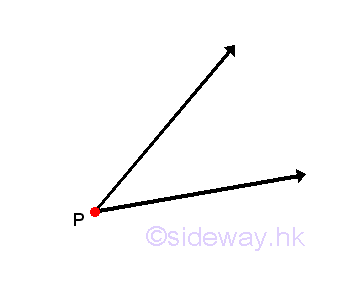Measure of Angle

The amount of angular rotation between the initial and terminal rays is measured by a number. In some typical case, the two rays may coincide with each other in the same direction then the angle measure is zero. Or  the two ray may be along the same line in opposite direction then the angle measure is a striaght angle. The initial ray can rotate either closewise or counter-clockwise to the position of the terminal ray. There are two ways to consider the rotation angle with positive angle is generated by counter-clockwise rotation and negative angle is generated by clockwise rotation. Although measure of an angle is usually in absolute value, oriented or signed angles, also callede directed, sensed angles, are also commonly used when the direction of rotation is important. ImplyDegree Measure of Angle

One common angle measuring unit is degree measure with notation "o". This is not a SI unit. But degree measure is a common accepted unit for the description of an angle numerically especially in trigonomety study. One degree unit is defined by dividing one complete revolution of the initial side back to the initial side by 360 parts. Therefore one complete revolution is equal to 360o and parts of a revolution are measured proportionately. Since the initial side coincides with the terminal side, a 0 degree rotation or no rotation at all is equal to a 360 degee rotation and a 360 degree rotation is same as a -360 degree rotation. A striaght angle is equal to one half of a complete revolution, that is (360o)/2=180o. As the location of terminal side is  the same, when the initial side rotates a half revolution in either clockwise or counter-clockwise direction, a 180 degree rotation is same as a -180 degree rotation. A quarter of a complete revolution is called a right angle is equal to (360o)/4=90o. For an angle which measures between 0o and 90o, is called an acute angle. For an angle which measures between 90o and 180o, is called an obtuse angle. When the sum of two angles is equal to 90o, the two angles are complementary angles. When the sum of two angles is equal to 180o, the two angles are supplementary angles. Angle measure less than one degree can be subdivide into decimal degrees or convertng to the Degree-Minute-Second (DMS) form. In the Degree-Minute-Second (DMS) system, one degree is divided into sixty minutes with notation " ' " equally, and one minute is further subdivided into sixty seconds with notation " " " equally also. That is 1o=60'=3600". Besides, for the represntation of rotational motion more than one revolution, angle measure can be more than 360o.

Angles on Coordinate Plane

In order to express an angle algebrically, an angle can be overlayed on the coordinate plane by locating the vertex of an angle on the origin and coinciding the initial side with the positive x-axis, that is in the standard position. Therefore, angle in standard position can be classfied according to the location of the terminal side of the angle, that is the four quadrants, I, II, III, and IV, of the coordinate plane. For example, angle with terminal side lies in quadrant I is called a quadrant I angle. And angle with terminal side lies on the coordinate axis is called quadrantal angle. When two standard angles are with the same initial and terminal sides, the two angles are coterminal angles. Since two angles share the same initial and terminal sides, the difference of the two coterminal angle is equal to 360o or -360o. Since the terminal side of an angle always repeats on a multiple of 360o, therefore two coterminal angles always differ by a multiple of 360o, that is difference of coterminal angles, α-β=n*(360o). As there are infinitely many integers n, a given angle also has infinitely many coterminal angels.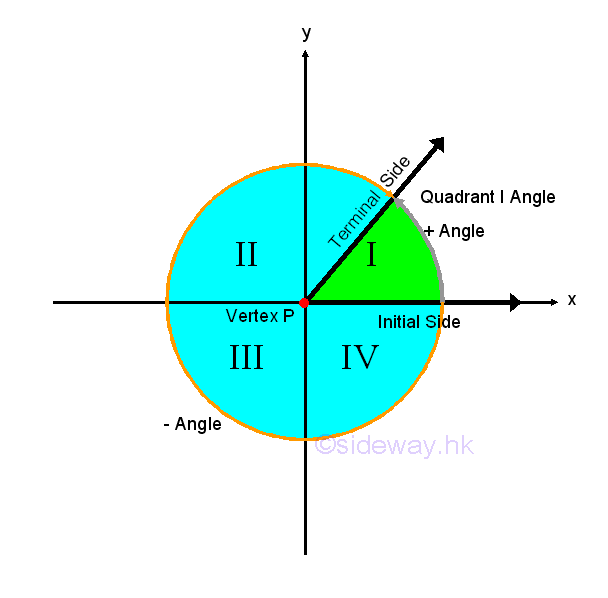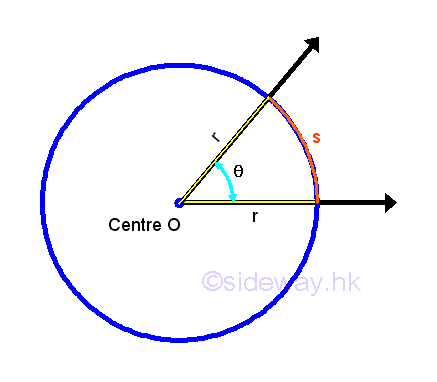Since the angle measure of one completer revolution is equal to 360 in degree  or 2π in radian, two measuring unit can be convert to each other throught a proportional ratio. Angle with a degree measure can be converted to a radian measure by multiplying a proportional ratio of 2π radian/360 degree=π radian/180o.  Or angle with a radian measure can be converted to a degree measure by multiplying a proportional ratio of  360 degree/2π radian=180o/π  radian.

Right-Angled Triangle

The definition of Trigonometric functions are based on the relationship between the sides and the angle of a right or right- angled triangle. A right angled triangle is a triangle with one angle is a right angle. The sides of a triangle are named according the location of the specified angle. Opposite side is located opposite to the specified angle θ. Hypotenuse side is the longest side of the triangle. Adjacent side is located next to or adjacent to the specified angle θ.Trigonometric Functions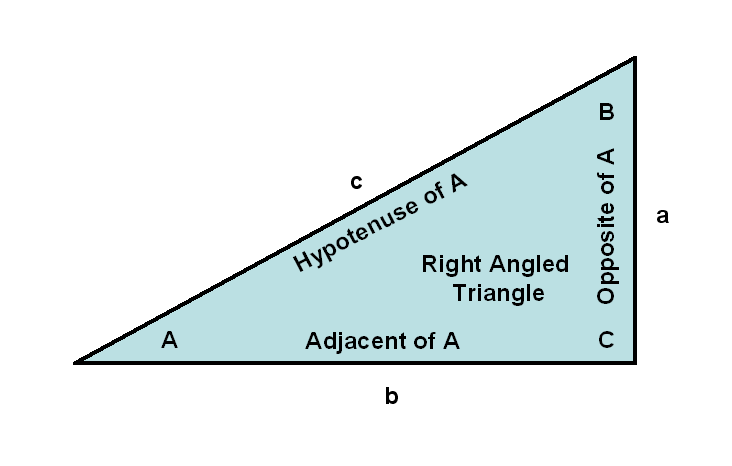The definition of the trigonometric functions based on a right or right- angled triangle are the length of one side divided by another side. The three main trigonometric functions are sine, cosine, and tangent functions. The sine function of A, denoted by sin A, is defined as the opposite side of A divided by the hypotenuse side of A.  ImplyThe cosine function of A, denoted by cos A, is defined as the adjacent side of A divided by the hypotenuse side of A. Imply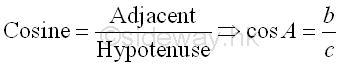And the tangent function of A, denoted by tan A, is defined as the opposite side of A divided by the adjacent side of A. The sine, cosine and tangent functions of B can also be obtained accordingly. ImplyThere are another three trigonometric functions which can be defined as the reciprocal functions of the above three functions correspondingly. The cosecant function of A, denoted by csc A, or cosec A, can be defined as the reciprocal of sine function of A and is equal to the hypotenuse side of A divided by the opposite side of A. ImplyThe secant function of A, denoted by sec A, can be defined as the reciprocal of cosine function of A and is equal to the hypotenuse side of A divided by the adjacent side of A. ImplyThe cotangent function of A, denoted by cot A, can be defined as the reciprocal of tangent function of A and is equal to the adjacent side of A divided by the opposite side of A. ImplyPythagorean theoremPythagorean theorem or Pythagoras' theorem is the theorem about the relationship of the lengths of the three sides of a right angled triangle which can be used to find the values of trigonometric functions for some typical angles. Assumed the two sides next to the right angle are legs of length a and b, and the third side is the hypotenuse of length c. The Pythagorean theorem states that the sum of the squares of the lengths of the two legs of a right triangle is equal to the square of the length of the hypotenuse. That is a2+b2=c2. Imply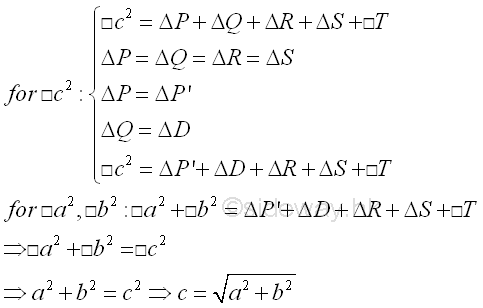Values of Trigonometric Functions

Values of trigonometric functions for some typical angles can be determined by making use of some special types of triangles and the Pythagorean theorem geometrically. An equilateral triangle is a triangle with three equal sides and therefore the internal angles are also congruent angles and are equal to 60o. Let the lengths of sides of the equilateral triangle equal to c. By constructing a vertical perpendicular bisector from the top vertex, the equilateral triangle is divided into two equal parts with the bottem side of the equilateral triangle is divided into two equal parts of the same length. Let the length of the vertical bisector be a and the length of the bisected sides be b, then c=2b. Since the perpendicular bisector coincides with the angle bisector, the top vertex angle is also bisected into two equal angles, that is 30o=60o/2. Imply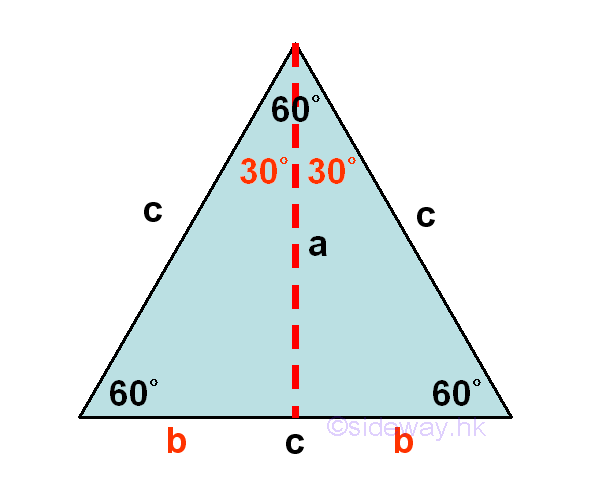Since b can be expressed in term of c, that is b=c/2, a can also be found in term of c by making use of the Pythagorean theorem, that is a2+b2=c2⇒a=√(c2-b2). Imply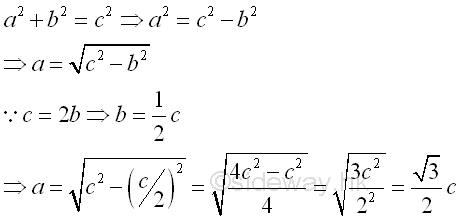Therefore the relationships of the sides of a right-angled triangle of 30-60-90 degrees can be obtained. And the proportions of the sides can also simplified to a:b:c=(√3/2)c:(1/2)c:c=√3:1:2. ImplyAs the lengths of the sides of the 30-60-90 triangle are known, values of trigonometric functions for 30o and 60o can be found accordingly.  Imply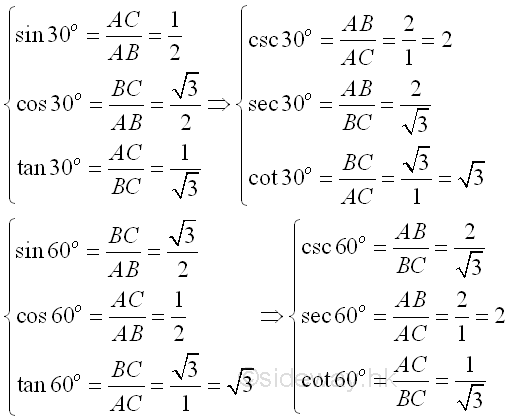Consider an isosceles right-angled triangle of 45-45-90 degrees with two equal sides. Let the legs of the two equal sides next to the right angle are a and the third side of the hypotenuse be c.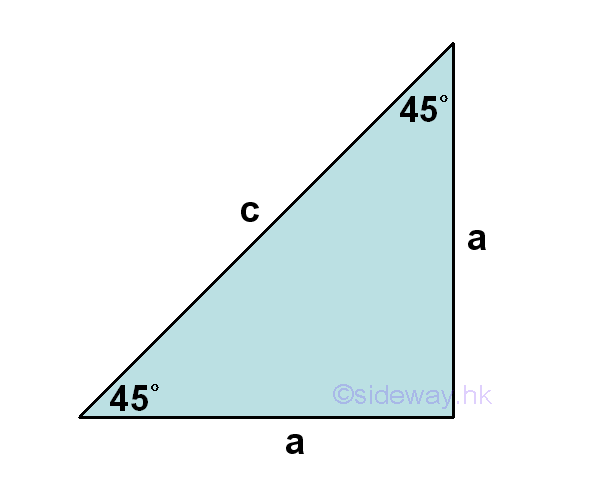Since the triangle is already a right-angled triangle, Pythagorean theorem can be applied directly, that is that is a2+a2=c2⇒c=√(a2+a2)=√(2a2)=(√2)a .  ImplyTherefore the relationships of the sides of a right-angled triangle of 45-45-90 degrees can be obtained. And the proportions of the sides can also simplified to a:a:c=a:a:(√2)a=1:1:√2. ImplyAs the lengths of the sides of the 45-45-90 triangle are known, values of trigonometric functions for 45o can be found accordingly.  ImplySimilarly, values of trigonometric functions for 0o  and 90o can also be found according to the definition of trigonometric function by constructing imaginary triangles, that is a triangle of zero height for 0o and a triangle of infinite height for 90o.  Imply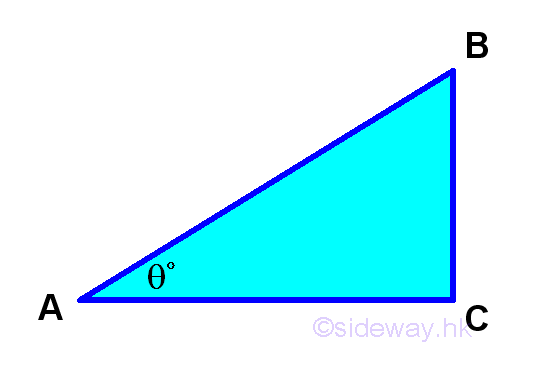Values of trigonometric functions for 0o  and 90o are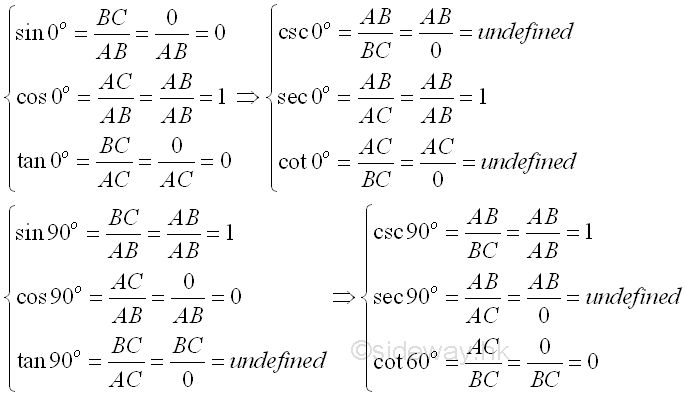Table of Trigonometric Functions for Common Angles

Degrees Radians sin θ cos θ tan θ cot θ sec θ csc θ
0 0 0 1 0 undefined 1 undefined
30 π/6 1/2 √3/2 √3/3 √3 2√3/3 2
45 π/4 √2/2 √2/2 1 1 √2 √2
60 π/3 √3/2 1/2 √3 √3/3 2 2√3/3
90 π/2 1 0 undefined 0 undefined 1
120 2π/3 √3/2 -1/2 -√3 -√3/3 -2 2√3/3
135 3π/4 √2/2 -√2/2 -1 -1 -√2 √2
150 5π/6 1/2 -√3/2 -√3/3 -√3 -2√3/3 2
180 π 0 -1 0 undefined -1 undefined
210 7π/6 -1/2 -√3/2 √3/3 √3 -2√3/3 -2
225 5π/4 -√2/2 -√2/2 1 1 -√2 -√2
240 4π/3 -√3/2 -1/2 √3 √3/3 -2 -2√3/3
270 3π/2 -1 0 undefined 0 undefined -1
300 5π/3 -√3/2 1/2 -√3 -√3/3 2 -2√3/3
315 7π/4 -√2/2 √2/2 -1 -1 √2 -√2
330 11π/6 -1/2 √3/2 -√3/3 -√3 2√3/3 -2
360 0 1 0 undefined 1 undefined

More Values of Trigonometric Functions

By making use of the 45-45-90 and 30-60-90 triangles, trigonometric functions for 15o and 75o can also be found.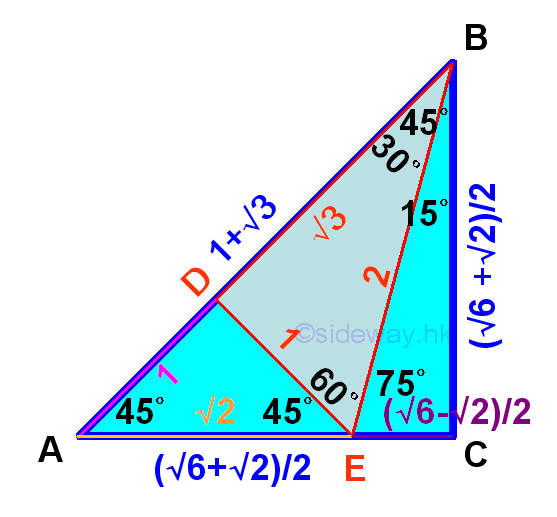Assume ED=AD=1 and DE=2, and AC=BC, then length of sides of triangles can be determined as following: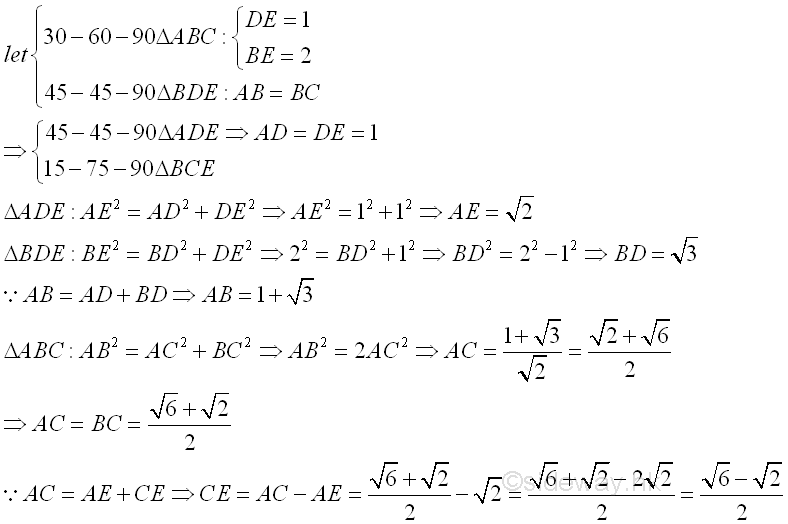Values of trigonometric functions for 15o  and 75o areID: 130500023 Last Updated: 6/27/2013 Revision: 2 Ref:References

1. B. Joseph, 1978, University Mathematics: A Textbook for Students of Science &amp; Engineering
2. Ayres, F. JR, Moyer, R.E., 1999, Schaum's Outlines: Trigonometry
3. Hopkings, W., 1833, Elements of TrigonometryHome 5

Management

HBR 3

Information

Recreation

Culture

Chinese 1097

English 337

Computer

Hardware 160

Software

Application 205

Latex 35

Manim 203

Numeric 19

Programming

Web 285

Unicode 504

HTML 65

CSS 65

SVG 46ASP.NET 270

OS 422

Python 71Knowledge

Mathematics

Algebra 84

Trigonometry 31

Geometry 32Calculus 67

Engineering

Mechanical

Rigid Bodies

Statics 92

Dynamics 37

Control

Natural Sciences

Electric 27